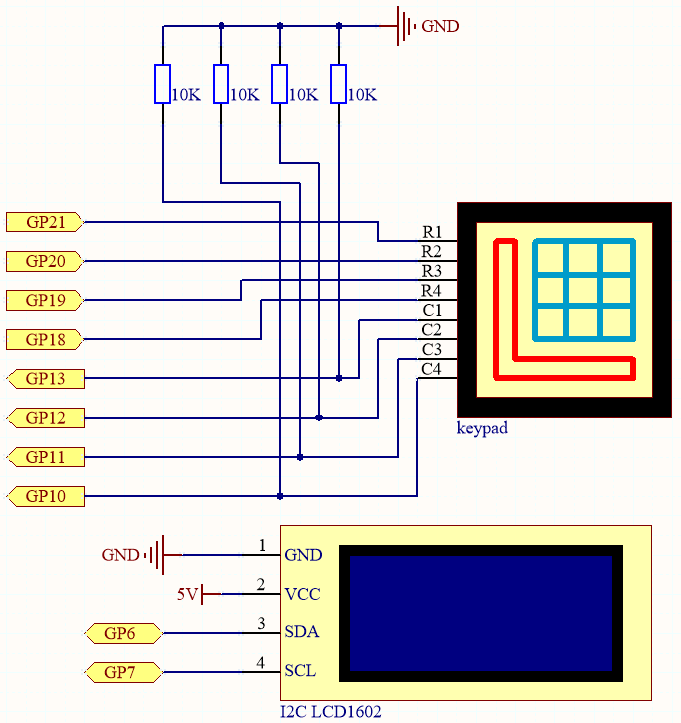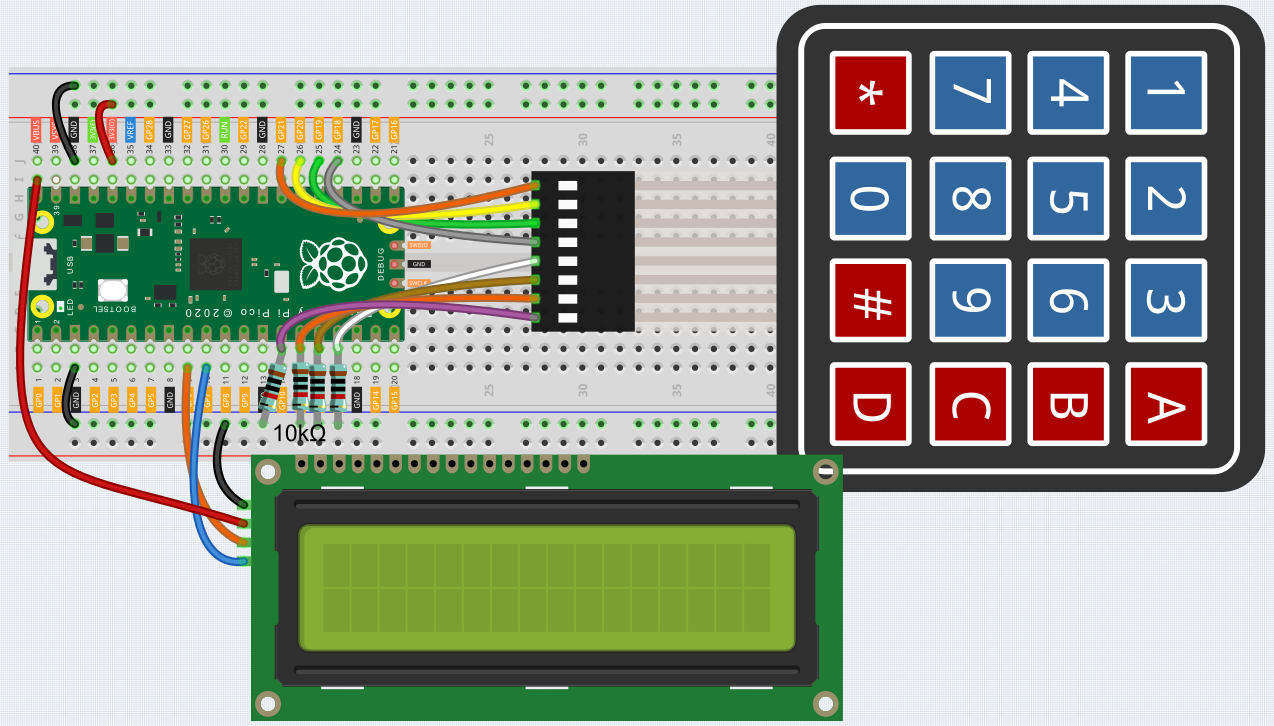# 7.7 Guess Number¶

Guessing Numbers is a fun party game where you and your friends input numbers (0-99). With each input of the number, the range will shrink until a player answers the riddle correctly. Then the player is defeated and punished.

As an example, if the lucky number is 51, which the players cannot see, and the player 1 inputs 50, the prompt changes to 50 - 99; if the player 2 inputs 70, the range changes to 50 - 70; if the player 3 inputs 51, the player is unlucky. In this case, numbers are inputted through the keypad, and outcomes are displayed on a LCD screen.

SchematicThis circuit is based on 4.2 4x4 Keypad with the addition of an I2C LCD1602 to display the pressed keys.

WiringTo make the wiring easier, in the above diagram, the column row of the matrix keyboard and the 10K resistors are inserted into the holes where G10 ~ G13 are located at the same time.

Code

Note

• Open the `7.7_game_guess_number.py` file under the path of `euler-kit/micropython` or copy this code into Thonny, then click “Run Current Script” or simply press F5 to run it.

• Don’t forget to click on the “MicroPython (Raspberry Pi Pico)” interpreter in the bottom right corner.

• For detailed tutorials, please refer to Open and Run Code Directly.

```from lcd1602 import LCD
import machine
import time
import urandom

characters = [["1","2","3","A"],["4","5","6","B"],["7","8","9","C"],["*","0","#","D"]]

pin = [21,20,19,18]
row = []
for i in range(4):
row.append(None)
row[i] = machine.Pin(pin[i], machine.Pin.OUT)

pin = [13,12,11,10]
col = []
for i in range(4):
col.append(None)
col[i] = machine.Pin(pin[i], machine.Pin.IN)

key = []
for i in range(4):
row[i].high()
for j in range(4):
if(col[j].value() == 1):
key.append(characters[i][j])
row[i].low()
if key == [] :
return None
else:
return key

# init/reset number
# reset the result as False for lcd show
def init_new_value():
global pointValue,upper,count,lower
pointValue = int(urandom.uniform(0, 99))
print(pointValue)
upper = 99
lower = 0
count = 0
return False

# lcd show message
# If target, show game over.
# If not target, or not detected, show guess number.
def lcd_show(result):
lcd.clear()
if result == True:
string ="GAME OVER!\n"
string +="Point is "+ str(pointValue)
else :
string ="Enter number: " + str(count) +"\n"
string += str(lower)+ " < Point < " + str(upper)
lcd.message(string)
return

# detect number & reflesh show message
# if not target, reflesh number (upper or lower) and return False
# if target, return True
def number_processing():
global upper,count,lower
if count > pointValue:
if count < upper:
upper = count
elif count < pointValue:
if count > lower:
lower = count
elif count == pointValue:
return True
count = 0
return False

## start
lcd = LCD()
string = "Welcome!\n"
string = "Press A to Start!"
lcd.message(string)
result=init_new_value()

last_key = None
while True:
if current_key == last_key:
continue
last_key = current_key
if current_key != None:
# print(current_key)
if current_key ==["A"]: # reset number
result=init_new_value()
elif current_key==["D"]: # check
result=number_processing()
elif current_key in list(["1","2","3","4","5","6","7","8","9","0"]) and count < 10: #check validity & limit digits
count = count * 10 + int(current_key)
lcd_show(result) # show
time.sleep(0.1)
```
• After the code runs, press `A` to start the game. A random number `point` is produced but not displayed on the LCD, and what you need to do is to guess it.

• The number you have typed appears at the end of the first line till the final calculation is finished. (Press `D` to start the comparation.)

• The number range of `point` is displayed on the second line. And you must type the number within the range.

• When you type a number, the range narrows; if you got the lucky number luckily or unluckily, there will appear `GAME OVER!`.

Note

If the code and wiring are fine, but the LCD still does not display content, you can turn the potentiometer on the back to increase the contrast.